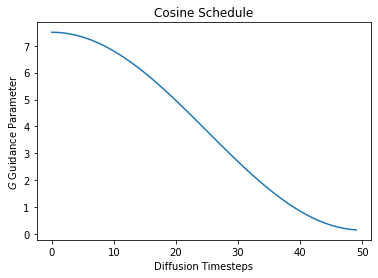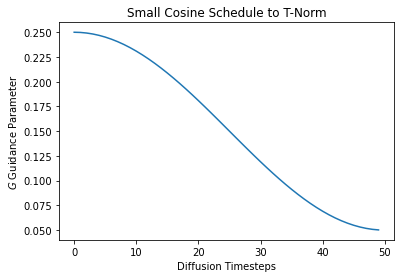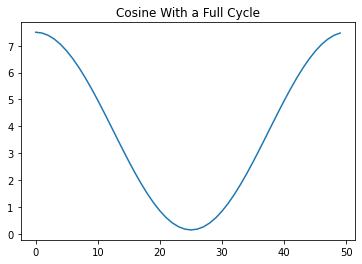# cf_guidance

This library has normalizations and schedules for the Classifier-free Guidance parameter in Diffusion models. We call the guidance modifiers transforms.

It seems that transforms can improve the quality of generated images across Diffusion pipelines. To be specific:

• Normalizing can help with image syntax.
• Scheduling can improve image details.

For more details, please check out the official blog post.

## Install

pip install cf_guidance


## How to use

The follow section creates a few example schedules. Then, we use the schedules to initialize different kinds of transforms.

### Creating Cosine schedules

We create a few different schedules:

A Cosine schedule that starts from the default Guidance parameter in diffusion $\left( G = 7.5\right)$ and works it way down to a minimum of $0.15$.

Next we create the same schedule, except with an initial warmup. With warmup, $G$ starts from $0.15$ and linearly works up to a maximum $G_\text{max} = 7.5$. Here we warmup over the first 10% of the total diffusion steps. After warmup, the schedule decreases following a cosine curve.

Finally, we create a schedule with small values of $G$. This is meant for the guidance transform TNormGuidance. This transforms puts the vectors on a similar scale, so we need a much smaller value than usual when mixing them.

from cf_guidance.schedules import get_cos_sched

# Parameters from the blog post
max_val = 7.5
min_val = 0.15
num_steps = 50
num_warmup_steps = 5

# 1) Baseline cosine schedule
cos_params = {
'max_val':           max_val,
'num_steps':         num_steps,
'min_val':           min_val,
'num_warmup_steps':  0,
}

# 2) Cosine schedule with warmup
warmup_cos_params = {
'max_val':           max_val,
'num_steps':         num_steps,
'min_val':           min_val,
'warmup_init_val':   min_val + 1., # to show we can offset the warmup relative to min
'num_warmup_steps':  num_warmup_steps,
}

# 3) Cosine schedule with small values for T-Norm guidance
t_scale_params = {
'max_val':         0.25,
'num_steps':       num_steps,
'min_val':         0.05,
}

# create the schedules
cos_g = get_cos_sched(**cos_params)
warmup_g = get_cos_sched(**warmup_cos_params)
TNorm_g = get_cos_sched(**t_scale_params)


Let’s plot these schedules to see what they look like.

import matplotlib.pyplot as plt

# plot them
plt.plot(cos_g)
plt.xlabel('Diffusion Timesteps')
plt.ylabel('$G$ Guidance Parameter')
plt.title('Cosine Schedule');plt.plot(warmup_g)
plt.xlabel('Diffusion Timesteps')
plt.ylabel('$G$ Guidance Parameter')
plt.title('Warmup Cosine Schedule');plt.plot(TNorm_g)
plt.xlabel('Diffusion Timesteps')
plt.ylabel('$G$ Guidance Parameter')
plt.title('Small Cosine Schedule to T-Norm');### Creating Guidance Transforms

Guidance transforms take one initialization parameter: schedules. This is a map from parameter names to an array-like, indexable sequence of values.

For a given parameter name at diffusion timestep idx, the value of schedules[name][idx] should be the value of that parameter at the given timestep.

In this case, we call the parameter $G$ as a lowercase ‘g’.

from cf_guidance.transforms import *

# Create a baseline Guidance with cosine schedule.
sample_sched = {'g': cos_g}
guidance = GuidanceTfm(sample_sched)

# Guidance with normalization and a cosine schedule.
norm_guidance = BaseNormGuidance(sample_sched)

# T-Norm Guidance with the smaller-valued schedule
small_sched = {'g': TNorm_g}
small_guidance = TNormGuidance(small_sched)


### Using the transforms in a Diffusion pipeline

The code snippet below shows where and how these transforms are used in an image generate() pipeline. We will use the norm_guidance class created above for the example.

Specifically, we call this norm_tfm with the following arguments:

• The unconditioned noise predictions.
• The conditional noise predictions.
• The current timestep index.

We borrow code from the official StableDiffusionPipeline from HuggingFace to show where our norm_tfm goes. This seems like a good starting point, since many scripts and functions are based on this HuggingFace script.

    # inside of StableDiffusionPipeline

for i, t in enumerate(self.progress_bar(timesteps_tensor)):
# expand the latents if we are doing classifier free guidance
latent_model_input = torch.cat([latents] * 2) if do_classifier_free_guidance else latents
latent_model_input = self.scheduler.scale_model_input(latent_model_input, t)

# predict the noise residual
noise_pred = self.unet(latent_model_input, t, encoder_hidden_states=text_embeddings).sample

# NOTE: our transforms go here:
###############################
if do_classifier_free_guidance:
noise_pred_uncond, noise_pred_text = noise_pred.chunk(2)

#noise_pred = noise_pred_uncond + guidance_scale * (noise_pred_text - noise_pred_uncond)

# NEW cf_guidance UPDATE
noise_pred = norm_guidance(noise_pred_uncond, noise_pred_text, i)


### Creating more cosine schedules

The cosine schedule function was based on a combination of the Cosine schedulers in timm and HuggingFace.

It has a variety of parameters to support many different schedule combinations, as shown below.

# using wilder parameters, worth exploring
two_cycles = {
'max_val':           max_val,
'num_steps':         num_steps,
'min_val':           min_val,
'num_cycles':        1,
'warmup_init_val':   min_val + 1., # to show we can offset the warmup relative to min
'num_warmup_steps':  0,
}

k_decay_cos = {
'max_val':           max_val,
'num_steps':         num_steps,
'min_val':           min_val,
'num_cycles':        1.5,
'k_decay':           0.7,
'warmup_init_val':   min_val + 1., # to show we can offset the warmup relative to min
'num_warmup_steps':  num_warmup_steps,
}

# create the schedules
two_cycle_sched = get_cos_sched(**two_cycles)
k_decay_sched = get_cos_sched(**k_decay_cos)

plt.plot(two_cycle_sched)
plt.title('Cosine With a Full Cycle');plt.plot(k_decay_sched)
plt.title('Cosine with Warmup, 1.5 Cycles, and K-decay');